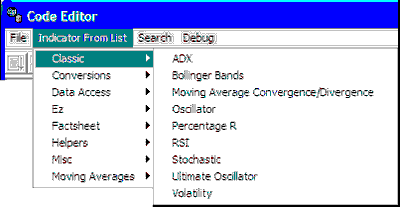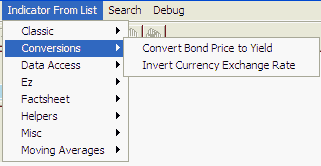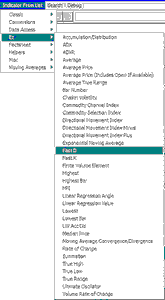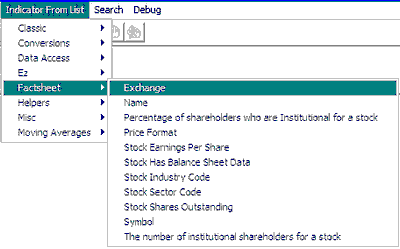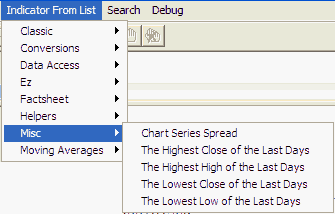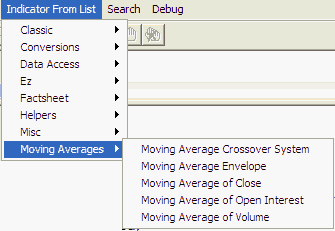Sample Study Lists Previous  Top  Next

The list of available studies and helpers may be slightly different for the different programming language. Each is also subject to change, either from CSI or through your own modifications. Here are some examples to give you an idea of what might be available to you.

Below is the list of indicators and helper programs:

·   Classic
 ·   BollingerBands(15,1.5) = 15-day Bollinger Bands
 ·   ChakinOscillator(3,10) = Chakin Oscillator
 ·   Macd(5,10,3) = 5,10,3-day Macd
 ·   Oscillator(5) = 5-day Oscillator
 ·   Percentage_R(5) = 5-day Percent R
 ·   RSI(5) = 5-day RSI
 ·   StocCross(5,3,3) = 5/3/3-day Stochastic Trigger
 ·   Stochastic(5,3,3) = 5/3/3-day Stochastic
 ·   Ultimate_Oscillator(7,14,28) = Ultimate Oscillator
 ·   Volatility(5) = 5-day Volatility

·   Conversions
 ·   InvertExchangeRate(14) = Inverts Price values
 ·   PriceToYield(2,\$Close) = The current (2Yr Note) close in Yield

·   Data Access
 ·   C(0) = Close
 ·   H(0) = High
 ·   I(0) = Open Interest
 ·   L(0) = Low
 ·   O(0) = Open
 ·   OHLCStudy() = Returns the current OHLC
 ·   V(0) = Volume

·   Examples
 ·   ComputeADOscillator1() = Example function users manual
 ·   ComputeADOscillator2() = Example function users manual
 ·   ComputeADOscillator3() = Example function users manual already documented in manual, perhaps you can reformat
 ·   DetrendedBollingerBands(15,1.5) = 15-day Detrended Bollinger Bands
 ·   FilterBollingerBands(15,1.5,3) = 15-day Bollinger Bands w/ 3-day MA filter
 ·   ModifiedBollingerBands(15,1.5) = 15-day Bollinger Bands based on ATR

·   Factsheet
 ·   BigPointValue() = Value in currency for a whole point move
 ·   GetCvf() = Price Format
 ·   GetDividendGrowth5Year() = 5 Year Dividend Growth
 ·   GetDividendYield() = Dividend Yield
 ·   GetEarnings() = Stock Earnings
 ·   GetEarningsPerShare() = Stock Current Earnings Per Share
 ·   GetEarningsPerShareTotalLastYear() = Earnings Per Share Total Last Year
 ·   GetExchange() = Exchange
 ·   GetHasStockFundamentalData() = Has Stock Fundamental Data
 ·   GetIncomeGrowth5Year() = Income Growth for 5 Years
 ·   GetIndustryCode() = Stock Industry Code
 ·   GetLatestAnnualOperatingRevenue() = Last Annual Operating Revenue
 ·   GetLatestAnnualTotalNetIncome() = Latest Annual Total Net Income
 ·   GetMarketCapital() = Stock Market Capital
 ·   GetName() = Name
 ·   GetPE5YearAverage() = PE5 Year Average
 ·   GetPercentageInstitutionalShareHolders() = Percentage of shares held by Institutions
 ·   GetPointValue() = Point Value
 ·   GetPriceEarningsRatio() = Price/Earnings Ratio
 ·   GetRevenueGrowth5Year() = 5 Year Revenue Growth
 ·   GetSectorCode() = Stock Sector Code
 ·   GetSharesOutstanding() = Stock Shares Outstanding
 ·   GetStockBeta() = 36 Week Stock Beta
 ·   GetSymbol() = Symbol
 ·   NumInstitutionalShareHolders() = The number of institutional shareholders for a stock

·   Helpers
 ·   AveragePrice(0) = 25% Close + 25% High + 25% Low + 25% Open
 ·   Average_True_Range(5) = 5-day Average True Range
 ·   AvgPrice(20) = AvgPrice
 ·   ChangeOfPriceEarnings(5) = Returns the change in the P/E cased by the change in price over the last period
 ·   TypicalPrice(0) = 33% Close + 33% High + 33% Low
 ·   VolumeRateOfChange(5) = Rate of Change of Volume over last 6 days
 ·   WeightedClose(0) = 50% Close + 25% High + 25% Low

·   Misc
 ·   HighestClose(5) = 5-day highest close
 ·   HighestHigh(5) = 5-day highest high
 ·   LowestClose(5) = 5-day lowest close
 ·   LowestLow(5) = 5-day lowest low

·   MoneyFlow
 ·   MoneyFlow(5) = 5-day avg of Moneyflow
 ·   MoneyFlow3(20) = MoneyFlow3
 ·   MoneyFlowAsNewspaper(200) = 200 - Day MoneyFlow as Newspaper

·   Moving Averages
 ·   EMA(14) = Exponential Moving Average
 ·   MACross(3,5) = 100*(MA3 - MA5)/(MA3 + MA5)
 ·   MAOfClose(5) = 5-day mov avg of close
 ·   MAOfOi(5) = 5-day mov avg of OI
 ·   MAOfVol(5) = 5-day mov avg of volume
 ·   SCTT
 ·   BullBearBalanceIndicator(20,30) = Daily Rise Indicator
 ·   CommodityChannelIndex(15) = 15-day Commodity Channel Index
 ·   CupAndHandle(4) = Cup and Handle Indicator
 ·   DailyRiseIndicator(5,25) = Daily Rise Indicator
 ·   EaseOfMovement = EaseOfMovement
 ·   EaseOfMovementEMA(5) = EMA of EaseOfMovement
 ·   FractalDimensionOfWaveforms(30) = Regularized Momentum Indicator
 ·   HPI(400,0.2) = HPI (Futures Only) where a one-cent move is \$400 and a smoothing of 2.0
 ·   HeikinAshi() = Heikin - Ashi Technique
 ·   HeikinAshiDiff() = Heikin - Ashi Diff Indicator
 ·   LWAccDis() = LWAccDis
 ·   LinearRegValue(25,3,@Close) = Linear Regression Prediction of Close 3 bars in future
 ·   LinearRegValueOfClose(25,3) = Linear Regression Prediction of Close 3 bars in future
 ·   MassIndex(9,25) = MassIndex
 ·   OnBalanceVol(50) = 50 - Day On Balance Volume
 ·   OpeningGapIndicator(5,25) = Opening Gap Indicator
 ·   Parabolic(0.01) = Parabolic
 ·   PriceVolumeTrend() = Price Volume Trend
 ·   RecentHighLowDate(5) = Most Recent New High's and New Low's
 ·   Regularized_EMA(14,9) = Regularized EMA Indicator
 ·   Regularized_Momentum(14,9) = Regularized Momentum Indicator
 ·   RevEngRSI_EMA(45,14) = RevEngRSI - EMA Indicator
 ·   RevEngRSI_SMA(45,14) = RevEngRSI - SMA Indicator
 ·   Swing\SwingHigh(1,1,20) = SwingHigh
 ·   Swing\SwingHighBar(1,1,20) = SwingHighBar
 ·   Swing\SwingIndex() = SwingIndex
 ·   Swing\SwingLow(1,1,20) = SwingLow
 ·   Swing\SwingLowBar(1,1,20) = SwingLowBar
 ·   TL\TLAngle(getClose(5),5,getClose(15),15) = TLAngle of line between close of 15 days ago and 5 days ago
 ·   TL\TLSlope(getClose(5),5,getClose(15),15) = Slope of line between close of 15 days ago and 5 days ago
 ·   TL\TLValue(getClose(5),5,getClose(15),15,0) = Extension of line between 5 and 15 days previous draw to current bar
 ·   Time and Implied Volatility and Volume\IIV_Lookback(29,25,252,50) = IIV_Lookback Indicator
 ·   Time and Implied Volatility and Volume\IIV_Thresholds(29,25,252,50) = IIV_Lookback Indicator
 ·   Time and Money Charts\TMChanLinesHi(25) = Chan Lines Hi Indicator
 ·   Time and Money Charts\TMChanLinesLo(25) = Chan Lines Lo Indicator
 ·   Time and Money Charts\TMStochMom(12,25,2) = Stochastic Momentum Indicator
 ·   VolAccDist(10) = 10 - Day Accumulation/Distribution of Volume

·   Unclassified
 ·   AtTheMoneyCallOptionVolume() = Call volume for the nears out of the money strike
 ·   EmergMkt(20,3) = EmergMkt 20/3
 ·   FiniteVolumeElement(0.003,22)
 ·   MovingAverageEnvelope(15,7) = 7% bands around the 15-day moving average

Study Examples in AnyLanguage